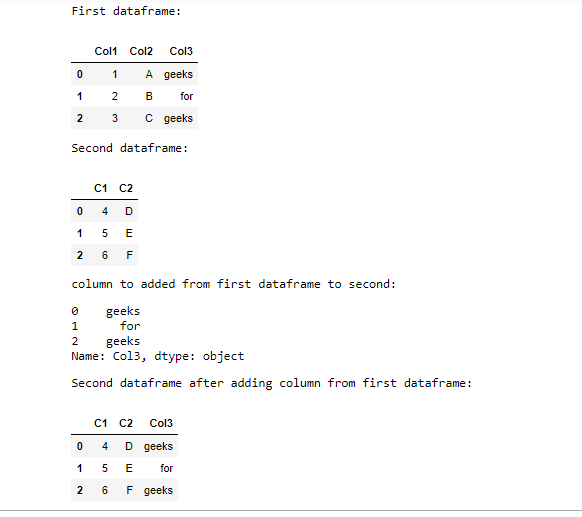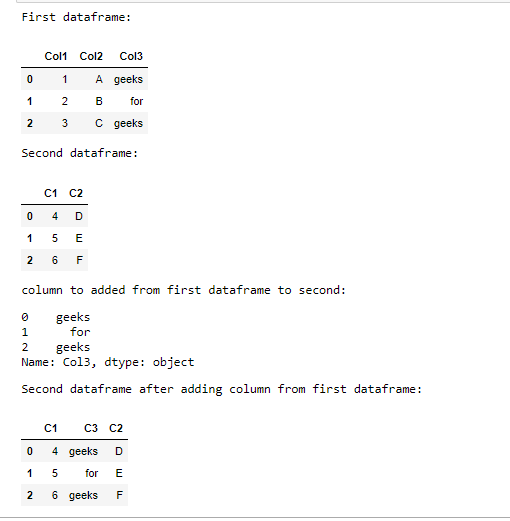Related Articles

# How to add column from another DataFrame in Pandas ?

• Last Updated : 30 May, 2021

Method 1: Using join()

Using this approach, the column to be added to the second dataframe is first extracted from the first using its name. Here the extracted column has been assigned to a variable.

Syntax: dataframe1[“name_of_the_column”]

After extraction, the column needs to be simply added to the second dataframe using join() function.

Syntax: Dataframe2.join(“variable_name”)

This function needs to be called with reference to the dataframe in which the column has to be added and the variable name which stores the extracted column name has to be passed to it as the argument. As a result, the column will be added to the end of the second dataframe with the same name as it was in the previous dataframe.

Example:

## Python3

 `import` `pandas as pd`` ` ` ` `df1 ``=` `pd.DataFrame({``"Col1"``: [``1``, ``2``, ``3``], ``                    ``"Col2"``: [``"A"``, ``"B"``, ``"C"``],``                    ``"Col3"``: [``"geeks"``, ``"for"``, ``"geeks"``]})`` ` `print``(``"First dataframe:"``)``display(df1)`` ` `df2 ``=` `pd.DataFrame({``"C1"``: [``4``, ``5``, ``6``],``                    ``"C2"``: [``"D"``, ``"E"``, ``"F"``]})`` ` `print``(``"Second dataframe:"``)``display(df2)`` ` `extracted_col ``=` `df1[``"Col3"``]``print``(``"column to added from first dataframe to second:"``)``display(extracted_col)`` ` `df2 ``=` `df2.join(extracted_col)``print``(``"Second dataframe after adding column from first dataframe:"``)``display(df2)`

Output:Method 2: Using insert()

The approach is the same as above- the column to be added is first extracted and assigned to a variable and then added to another dataframe. The difference here is that this approach gives freedom to place the column anywhere and with a different column name if need be.

Syntax: insert(location, “new_name”, “extarcted_column” )

Here, the index where the column is desired to inserted is passed in place of location. new_name can be replaced by the name the column is supposed to be renamed with and extracted_column is the column from the first dataframe.

Example:

## Python3

 `import` `pandas as pd`` ` ` ` `df1 ``=` `pd.DataFrame({``"Col1"``: [``1``, ``2``, ``3``],``                    ``"Col2"``: [``"A"``, ``"B"``, ``"C"``],``                    ``"Col3"``: [``"geeks"``, ``"for"``, ``"geeks"``]})``print``(``"First dataframe:"``)``display(df1)`` ` `df2 ``=` `pd.DataFrame({``"C1"``: [``4``, ``5``, ``6``],``                    ``"C2"``: [``"D"``, ``"E"``, ``"F"``]})``print``(``"Second dataframe:"``)``display(df2)`` ` `extracted_col ``=` `df1[``"Col3"``]``print``(``"column to added from first dataframe to second:"``)``display(extracted_col)`` ` `df2.insert(``1``, ``"C3"``, extracted_col)``print``(``"Second dataframe after adding column from first dataframe:"``)``display(df2)`

Output:Attention geek! Strengthen your foundations with the Python Programming Foundation Course and learn the basics.

To begin with, your interview preparations Enhance your Data Structures concepts with the Python DS Course. And to begin with your Machine Learning Journey, join the Machine Learning – Basic Level Course

My Personal Notes arrow_drop_up